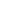# Free CBSE Class 9 Mathematics Unit 4-Geometry Quadrilaterals Worksheets

## Sample CBSE Class 9 Mathematics Unit 4-Geometry Quadrilaterals Worksheet Questions

1.

In the figure below, ABCD is a trapezium in which AB DC, BD is a diagonal and E is the mid - point of AD. A line is drawn through E parallel to AB intersecting BC at F. Which of the following statement is true?1. BC = 2BF 2. BC = 3BF/2 3. BC = 3BF 4. None of these
2.

ABCD is a quadrilateral and AP and DP are bisectors of and . The value of x is ________.1. 2. 3. 4.
3.

In the given figure, if ABCD is a parallelogram, then the value of is1. 2. 3. 4.
4.

In the given figure ABCD is a rhombus such that BD = 8 cm, find the length of BC.1. 2. 3. 4.
5.

In the figure, PQRS is a parallelogram in which , is equal to ______.1. 2. 3. 4.
6.

In a parallelogram ABCD, E and F are the mid-points of sides AB and CD respectively  given in the figure. Which of the following statement is true?1. DP = DB/2 2. DP = DB/3 3. DP = 2DB/3 4. DP = DB/4
7.

Chooae the correct option:  In the given figure, ABCD is a parallelogram and AP, BP, CR and DR are the angle bisectors of respectively. Then, PQRS is _______.1. Rhombus 2. Square 3. Rectangle 4. parallelogram
8.

Chooae the correct option:  In the given figure ABCD is a parallelogram where AP and CQ are the bisectors of  and . Then APCQ is a _______.1. Rhombus 2. Square 3. Rectangle 4. Parallelogram
9.

In the figure, ABCD is a rhombus whose side AB is produced to points P and Q such that AP = AB = BQ.  PD and QC are produced to meet at a point R.  What is the value of ?1. 2. 3. 4.
10.

In the figure, PQRS is a parallelogram whose diagonals intersect at O. Find the values of x.1. 2. 3. 4.

Worksheets by UrbanPro

Our worksheets are designed to help students explore various topics, practice skills and enrich their subject knowledge, to improve their academic performance. Designed by Experts who have extensive experience and expertise in teaching a subject, these worksheets will improve your child's problem-solving skills and subject knowledge in a fun and interactive manner.
Check out our free customized worksheets across school boards, grades, subjects and levels of subject knowledge. You can download, print and share these worksheets with anyone, anywhere, anytime!

Get a custom worksheet to practice!

Select your topic & see the magic.

subjectSelect Chapter(s)

Chapters & Subtopics